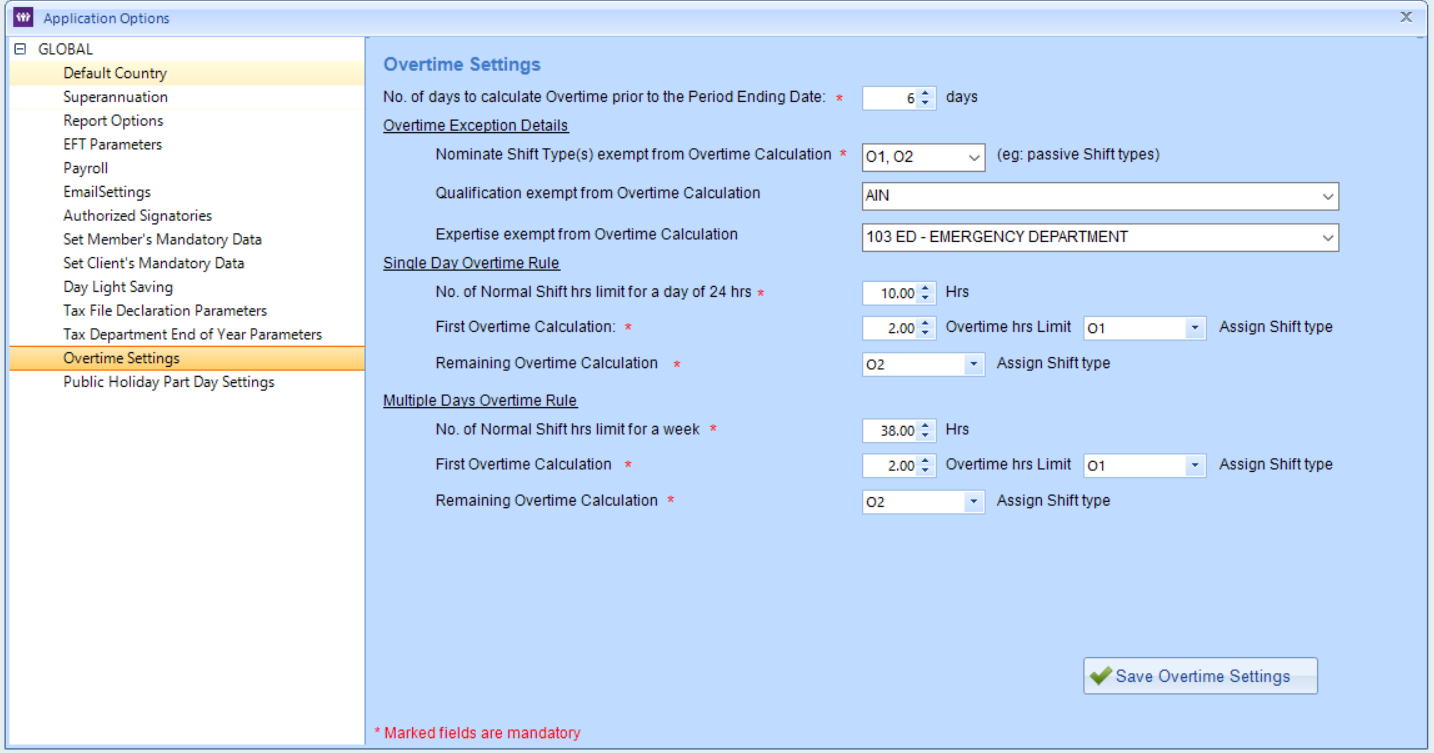### Overtime

In simple words, Overtime is the time worked in addition to one's normal working hours.

EntireHR is capable to handle your company's complete Overtime calculations.

To setup overtime settings in the system, Go to Tools menu in Finance module and select Application options, expand the Global settings and
select Overtime settings.No. of days to calculate Overtime prior to the Period Ending Date -  When we click on Overtime in the Main tool bar, the below window pops up before we can enter overtime section. Here you can notice that once you select the Period ending date the system automatically selects the from date. This from date is calculate on the basis of setting we define here.Overtime exception details -
under this area we define all the aspects of overtime calculation that we want to be an exception. Meaning system will not calculate overtime for details entered here.

Nominate shift(s) type exempt from overtime calculation - this drop down shows all the shift types available in the system and you have to select all those types for which you do not want overtime calculations.

Qualification exempt for overtime calculation - if you need to specify qualifications that you want to be exempted from overtime calculation, select them from this drop down.

Expertise exempt for overtime calculation - if you need to specify expertise that you want to be exempted from overtime calculation, select them from this drop down.

Single Day Overtime Rule -
under this area we define the rules for Single day overtime calculation. System needs to know when to calculate overtime and what will be the nominated shift type when overtime is calculated.

No. of Normal shift hours limit for a day of 24 hrs - Enter normal hours here in the total of 24 hrs a day for system to treat any worked hours on a day exceeding this limit as overtime hours. For ex. First Worked hrs on a day is mentioned as 10 hrs in the above sample.

First overtime calculation - this is where we mention the number of hours to be treat as overtime hrs after exceeding the normal hrs limit. For ex. 2 hrs mentioned above after 10 hrs of work on a day should be treated as O1.

Overtime hrs limit - Specify Overtime Shift Type for which you need to apply rate for the first overtime calculation. For ex. O1 is the shift type mentioned above.

Remaining Overtime Calculation - Specify Overtime Shift Type for which you need to apply rate for the remaining worked hrs after first overtime calculation hrs. For ex. O2 is the shift type mentioned above.

Multiple Day Overtime Rule -
Under this area we define the rules for Weekly overtime calculation. System needs to know when to calculate overtime and what will be the nominated shift type when overtime is calculated.

No. of Normal shift hours limit for a week: Monday 00:00 to Sunday 00:00- Enter normal hours here for system to treat any worked hours in a week exceeding this limit as overtime hours. For ex. First Worked hrs in a week is mentioned as 38 hrs in the above sample.

First overtime calculation - this is where we mention the number of hours to be treat as overtime hrs after exceeding the normal hrs limit. For ex. 2 hrs mentioned above after 38 hrs of work in a week should be treated as O1.

Overtime hrs limit - Specify Overtime Shift Type for which you need to apply rate for the first overtime calculation. For ex. O1 is the shift type mentioned above.

Remaining Overtime Calculation - Specify Overtime Shift Type for which you need to apply rate for the remaining worked hrs after first overtime calculation hrs. For ex. O2 is the shift type mentioned above.